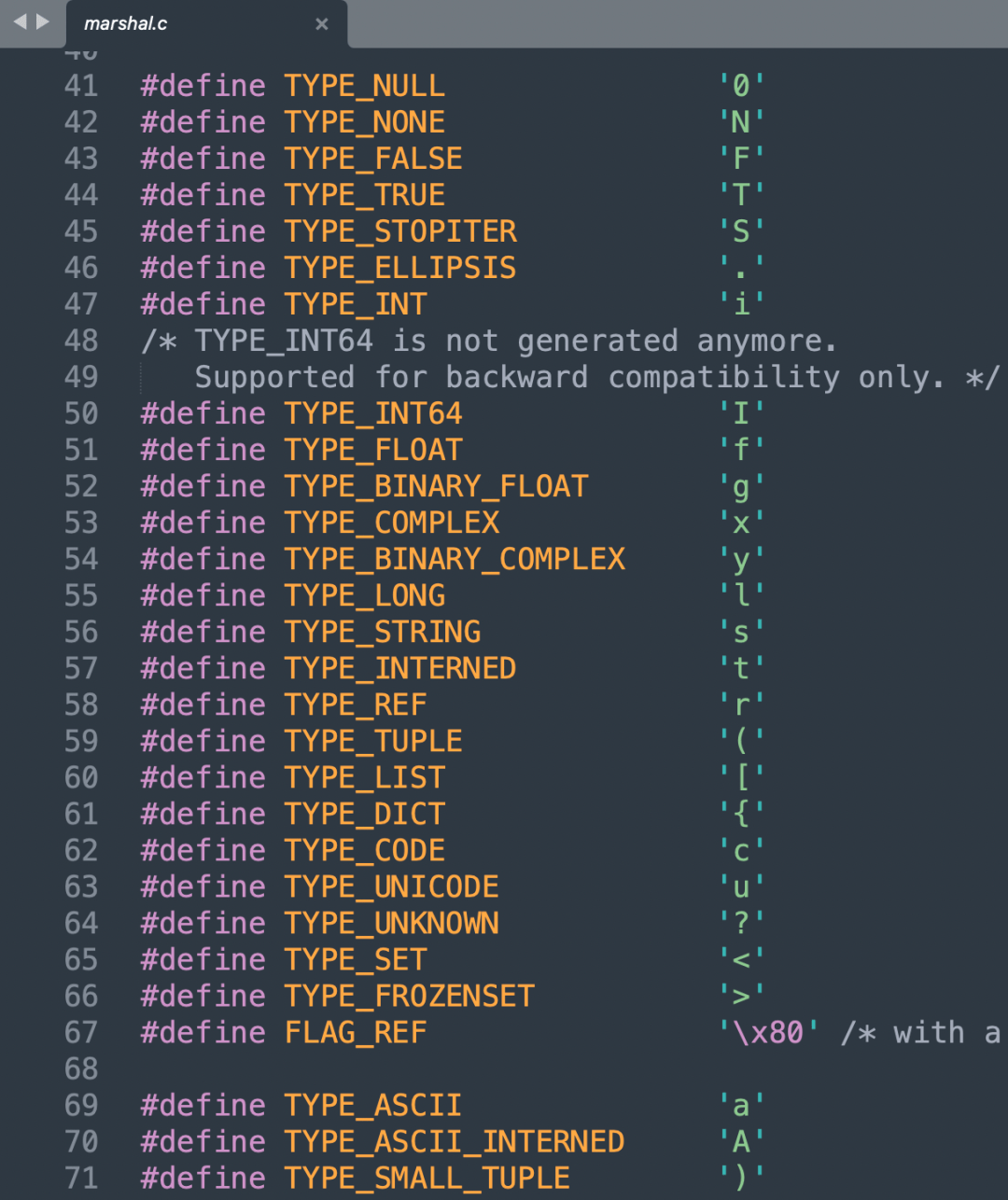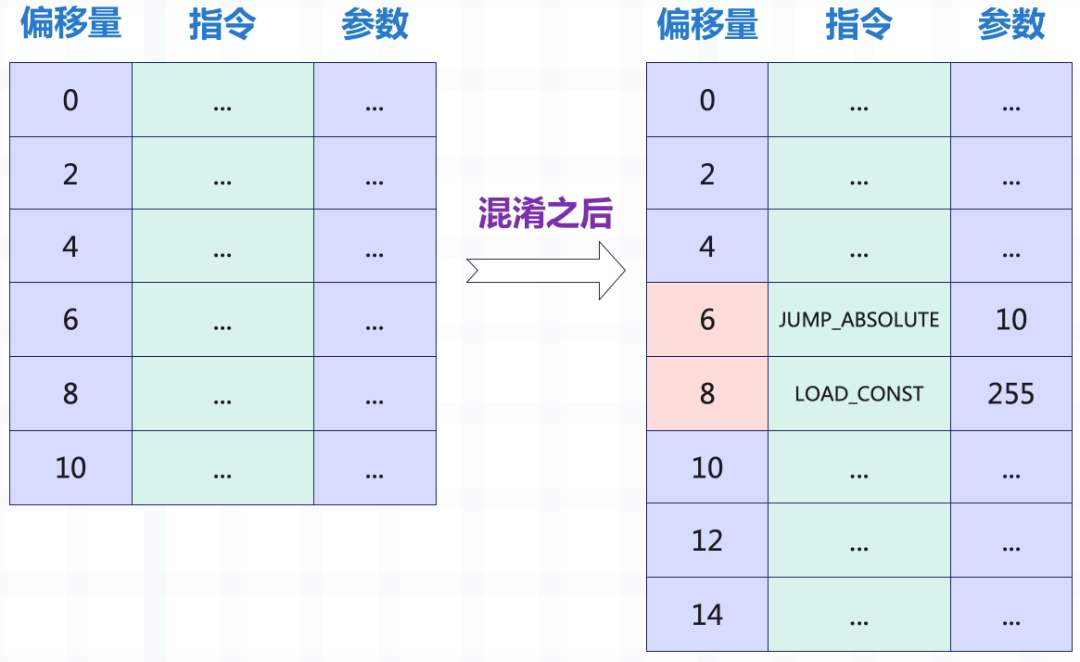# Python Pyc 文件解析：让你轻松理解 Python 编译后的字节码

``````a = 1
b = "你好啊"``````

``````import py_compile

py_compile.compile("tools.py")``````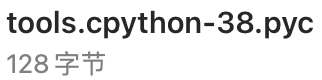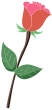pyc 文件的导入``````from importlib.machinery import SourcelessFileLoader

"tools", "__pycache__/tools.cpython-38.pyc"

print(tools.a)  # 1
print(tools.b)  # 你好啊``````pyc 文件包含的内容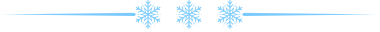pyc 文件在创建的时候都会往里面写入哪些内容呢？

1. magic number

2. pyc 文件的写入时间

3. py 文件的大小

py 文件的大小也会被记录在 pyc 文件中。

4. PyCodeObject 对象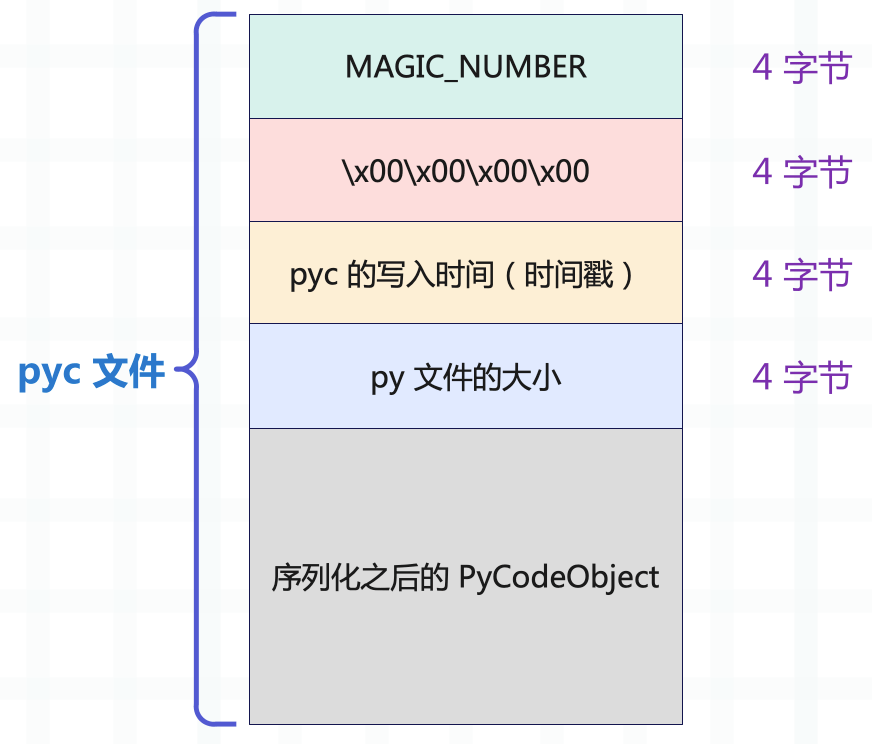``````import struct
from importlib.util import MAGIC_NUMBER
from datetime import datetime

with open("__pycache__/tools.cpython-38.pyc", "rb") as f:

# 0 ~ 4 字节是 MAGIC NUMBER
print(data[: 4])  # b'U\r\r\n'
print(MAGIC_NUMBER)  # b'U\r\r\n'

# 4 ~ 8 字节是 4 个 \x00
print(data[4: 8])  # b'\x00\x00\x00\x00'

# 8 ~ 12 字节是 pyc 的写入时间（小端存储），一个时间戳
ts = struct.unpack("<I", data[8: 12])
print(ts)  # 1671001724
print(
datetime.fromtimestamp(ts)
)  # 2022-12-14 20:32:23

# 12 ~ 16 字节是 py 文件的大小
print(
struct.unpack("<I", data[12: 16])
)  # 21``````

16 个字节往后就是 PyCodeObject 对象，并且是序列化之后的，因为该对象显然无法直接存在文件中。

``````import marshal

with open("__pycache__/tools.cpython-38.pyc", "rb") as f:

# marshal.dumps 则表示序列化
# 此时就拿到了 py 文件编译之后的 PyCodeObject
print(code)
"""
<code object <module> at 0x..., file "tools.py", line 1>
"""
# 查看常量池
print(code.co_consts)  # (1, '你好啊', None)

# 符号表
print(code.co_names)  # ('a', 'b')``````

``````a = 1
b = 2
c = 3``````

``````from importlib.util import MAGIC_NUMBER
import struct
import time
from types import CodeType
import marshal
from opcode import opmap

HEADER = MAGIC_NUMBER + b"\x00" * 4
# 时间随便写
# 大小随便写

# 构建 PyCodeObject
code = CodeType(
0,                # co_argcount
0,                # co_posonlyargcount
0,                # co_kwonlyargcount
3,                # co_nlocals
1,                # co_stacksize
0,                # co_flags

bytes([
# a = 1 分为两步
# 表示加载常量池中索引为 0 的常量
# 第二步：通过 STORE_NAME 将常量和符号绑定起来
# 参数是 0，表示和符号表中索引为 0 的符号进行绑定
opmap["STORE_NAME"], 0,
# b = 2
opmap["STORE_NAME"], 1,
# c = 3
opmap["STORE_NAME"], 2,
opmap["RETURN_VALUE"]
]),               # co_code

(1, 2, 3, None),  # co_consts
("a", "b", "c"),  # co_names
(),               # co_varnames
"build_pyc.py",   # co_filename
"<module>",       # co_name
1,                # co_firstlineno
b"",              # co_lnotab
(),               # freevars
()                # cellvars
)

# pyc 文件内容
# 生成 pyc 文件
with open("build_pyc.pyc", "wb") as f:
f.write(pyc_content)

# 然后加载生成的 pyc 文件
"build_pyc", "build_pyc.pyc"

print(mod)  # <module 'build_pyc' from 'build_pyc.pyc'>
print(mod.a)  # 1
print(mod.b)  # 2
print(mod.c)  # 3``````pyc 文件的写入``````//位置：Python/marshal.c

//FILE是 C 自带的文件句柄
//可以把WFILE看成是FILE的包装
typedef struct {
FILE *fp;  //文件句柄
//下面的字段在写入信息的时候会看到
int error;
int depth;
PyObject *str;
char *ptr;
char *end;
char *buf;
_Py_hashtable_t *hashtable;
int version;
} WFILE;``````

``````void
PyMarshal_WriteLongToFile(long x, FILE *fp, int version)
{
//magic number、创建时间和文件大小，只是一个整数
//在写入的时候，使用char 来保存
char buf;
//声明一个WFILE类型变量wf
WFILE wf;
//内存初始化
memset(&wf, 0, sizeof(wf));
//初始化内部成员
wf.fp = fp;
wf.ptr = wf.buf = buf;
wf.end = wf.ptr + sizeof(buf);
wf.error = WFERR_OK;
wf.version = version;
//调用w_long将x、也就是版本信息或者时间写到wf里面去
w_long(x, &wf);
//刷到磁盘上
w_flush(&wf);
}``````

``````static void
w_long(long x, WFILE *p)
{
w_byte((char)( x      & 0xff), p);
w_byte((char)((x>> 8) & 0xff), p);
w_byte((char)((x>>16) & 0xff), p);
w_byte((char)((x>>24) & 0xff), p);
}``````

w_long 则是调用 w_byte 将 x 逐个字节地写到文件里面去。

``````static void
w_object(PyObject *v, WFILE *p)
{
char flag = '\0';

p->depth++;

if (p->depth > MAX_MARSHAL_STACK_DEPTH) {
p->error = WFERR_NESTEDTOODEEP;
}
else if (v == NULL) {
w_byte(TYPE_NULL, p);
}
else if (v == Py_None) {
w_byte(TYPE_NONE, p);
}
else if (v == PyExc_StopIteration) {
w_byte(TYPE_STOPITER, p);
}
else if (v == Py_Ellipsis) {
w_byte(TYPE_ELLIPSIS, p);
}
else if (v == Py_False) {
w_byte(TYPE_FALSE, p);
}
else if (v == Py_True) {
w_byte(TYPE_TRUE, p);
}
else if (!w_ref(v, &flag, p))
w_complex_object(v, flag, p);

p->depth--;
}``````

w_complex_object 这个函数的源代码很长，我们看一下整体结构，具体逻辑就不贴了，后面会单独截取一部分进行分析。

``````static void
w_complex_object(PyObject *v, char flag, WFILE *p)
{
Py_ssize_t i, n;
//如果是整数的话，执行整数的写入逻辑
if (PyLong_CheckExact(v)) {
//......
}
//如果是浮点数的话，执行浮点数的写入逻辑
else if (PyFloat_CheckExact(v)) {
if (p->version > 1) {
//......
}
else {
//......
}
}
//如果是复数的话，执行复数的写入逻辑
else if (PyComplex_CheckExact(v)) {
if (p->version > 1) {
//......
}
else {
//......
}
}
//如果是字节序列的话，执行字节序列的写入逻辑
else if (PyBytes_CheckExact(v)) {
//......
}
//如果是字符串的话，执行字符串的写入逻辑
else if (PyUnicode_CheckExact(v)) {
if (p->version >= 4 && PyUnicode_IS_ASCII(v)) {
//......
}
else {
//......
}
}
else {
//......
}
}
//如果是元组的话，执行元组的写入逻辑
else if (PyTuple_CheckExact(v)) {
//......
}
//如果是列表的话，执行列表的写入逻辑
else if (PyList_CheckExact(v)) {
//......
}
//如果是字典的话，执行字典的写入逻辑
else if (PyDict_CheckExact(v)) {
//......
}
//如果是集合的话，执行集合的写入逻辑
else if (PyAnySet_CheckExact(v)) {
//......
}
//如果是PyCodeObject对象的话
//执行PyCodeObject对象的写入逻辑
else if (PyCode_Check(v)) {
//......
}
//如果是Buffer的话，执行Buffer的写入逻辑
else if (PyObject_CheckBuffer(v)) {
//......
}
else {
W_TYPE(TYPE_UNKNOWN, p);
p->error = WFERR_UNMARSHALLABLE;
}
}``````

``````static void
w_complex_object(PyObject *v, char flag, WFILE *p)
{
//......
else if (PyList_CheckExact(v)) {
W_TYPE(TYPE_LIST, p);
n = PyList_GET_SIZE(v);
W_SIZE(n, p);
for (i = 0; i < n; i++) {
w_object(PyList_GET_ITEM(v, i), p);
}
}
else if (PyDict_CheckExact(v)) {
Py_ssize_t pos;
PyObject *key, *value;
W_TYPE(TYPE_DICT, p);
/* This one is NULL object terminated! */
pos = 0;
while (PyDict_Next(v, &pos, &key, &value)) {
w_object(key, p);
w_object(value, p);
}
w_object((PyObject *)NULL, p);
}
//......
}``````

``````def foo():
lst = [1, 2, 3]

# 把列表内的元素写进去了
print(
foo.__code__.co_consts
)  # (None, 1, 2, 3)``````